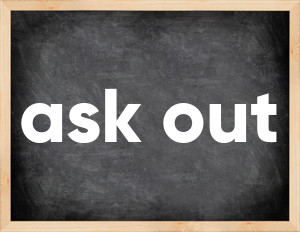The English verb 'ask out' is pronounced as [ɑːsk aʊt].
Related to: phrasal verb.

## Here are the past tense forms of the verb ask out

👉 Forms of verb ask out in future and past simple and past participle.
❓ What is the past tense of ask out.

## Ask out: Past, Present, and Participle Forms

Base Form Past Simple Past Participle

## What are the 2nd and 3rd forms of the verb ask out?

🎓 What are the past simple, future simple, present perfect, past perfect, and future perfect forms of the base form (infinitive) 'ask out'?

### Learn the three forms of the English verb 'ask out'

• the first form (V1) is 'ask out' used in present simple and future simple tenses.
• the second form (V2) is 'asked out' used in past simple tense.
• the third form (V3) is 'asked out' used in present perfect and past perfect tenses.

## What are the past tense and past participle of ask out?

The past tense and past participle of ask out are: ask out in past simple is asked out, and past participle is asked out.

### What is the past tense of ask out?

The past tense of the verb "ask out" is "asked out", and the past participle is "asked out".

### Verb Tenses

Future simple — ask out in future simple is ask out (will + V1).
Present Perfect — ask out in present perfect tense is asked out (have/has + V3).

### ask out regular or irregular verb?

👉 Is 'ask out' a regular or irregular verb? The verb 'ask out' is .

## Examples of Verb ask out in Sentences

•   to ask out to dinner (Present Simple)
•   a pupil asked out (Past Simple)
•   the dog is asking out (Present Simple)
•   Nine teachers asked out (Past Simple)
•   He called to ask out Jane and Daisy (Past Simple)

Along with ask out, words are popular chicken out and pencil.

Verbs by letter: , , , , , , , , , , , , , , , , , , , , , , , , .